Home    |    Teacher    |    Parents    |    Glossary    |    About UsBefore you evaluate an algebraic expression, you need to simplify it. This will make all your calculations much easier. Here are the basic steps to follow to simplify an algebraic expression:

1. remove parentheses by multiplying factors
2. use exponent rules to remove parentheses in terms with exponents
3. combine like terms by adding coefficients
4. combine the constants

Let's work through an example.When simplifying an expression, the first thing to look for is whether you can clear any parentheses. Often, you can use the distributive property to clear parentheses, by multiplying the factors times the terms inside the parentheses. In this expression, we can use the distributive property to get rid of the first two sets of parentheses.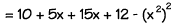Now we can get rid of the parentheses in the term with the exponents by using the exponent rules we learned earlier. When a term with an exponent is raised to a power, we multiply the exponents, so (x2)2 becomes x4.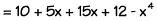The next step in simplifying is to look for like terms and combine them. The terms 5x and 15x are like terms, because they have the same variable raised to the same power -- namely, the first power, since the exponent is understood to be 1. We can combine these two terms to get 20x.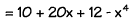Finally, we look for any constants that we can combine. Here, we have the constants 10 and 12. We can combine them to get 22.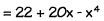Now our expression is simplified. Just one more thing -- usually we write an algebraic expression in a certain order. We start with the terms that have the largest exponents and work our way down to the constants. Using the commutative property of addition, we can rearrange the terms and put this expression in correct order, like this.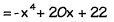Homework Help | Algebra | The Basics of AlgebraEmail this page to a friendSearch·  Properties of     Real Numbers·  Exponents·  Evaluating expressions·  Like terms·  SimplifyingFirst Glance In Depth Examples WorkoutSimplifying